# 吴恩达Stanford机器学习公开课（八）笔记

## Lecture 8 - Kernels.SMO algorithm.

Posted by Yunlongs on February 11, 2019

# Lecture 8 - Kernels.SMO algorithm.

## 核(Kernels)

SVM算法使用的是特征φ(x)，因此我们将在所有地方都使用φ(x)而不再是x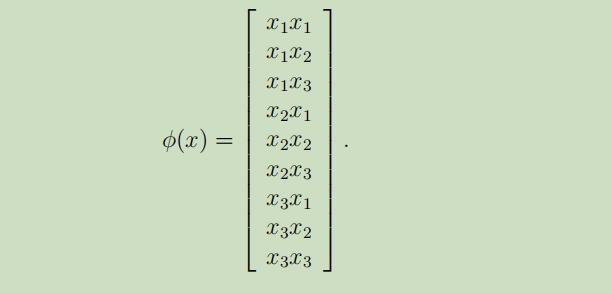注意，即使计算高维向量需要$O(n^2)$的时间复杂度，但是根据输入属性的线性，找到K(x,z)只需要$O(n)$的时间复杂度.

## 线性不可分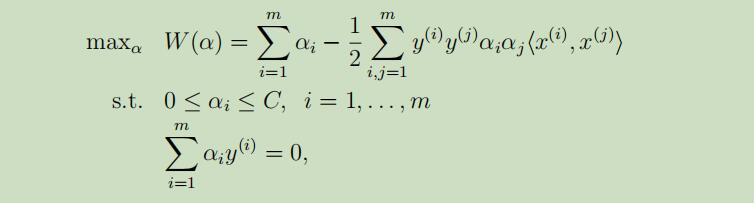## SMO（sequential minimal optimization）算法

### SMO算法

SMO算法是坐标上升算法的在SVM算法应用中的改进版，首先回顾我们需要解决的对偶优化问题：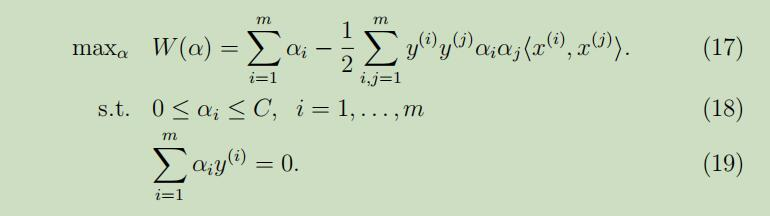在这里不同于上文讲的坐标上升算法的地方在于，需要满足限制条件(18-19)，如果我们保持除了α1外的参数固定来更新α1的话，将会出现如下问题： $\alpha_{1} y^{(1)}=-\sum_{i=2}^{m} \alpha_{i} y^{(i)}$ 当α2…αm都是固定的值时，α1在满足限制条件(18-19)的情况下也是个固定的值，因此我们不可能对α1进行更新。 假设现在令$\alpha_{3}, \dots, \alpha_{m}$固定，使用两个参数αi和αj来最优化W(x)，从限制条件19中我们可以得出如下等式： $\alpha_{1} y^{(1)}+\alpha_{2} y^{(2)}=-\sum_{i=3}^{m} \alpha_{i} y^{(i)}$ 因为等式右边都是固定的值，这里我们将其表示为常量$\zeta$：$\alpha_{1} y^{(1)}+\alpha_{2} y^{(2)}=\zeta$ 现在我们可以把关于α1和α2的限制条件在坐标系中显示出来，如下：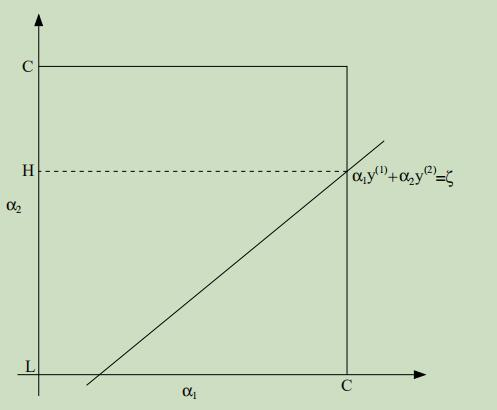从限制条件18可知，参数α1和α2必须落在如图的实线正方形中，图中的斜线段正是两个参数必须落下的地方以满足限制条件19。接着我们可以让上面的等式用α2来表达α1： $\alpha_{1}=(\zeta-\alpha_{2} y^{(2)}) y^{(1)}$ （$y^{(1)} \in\lbrace -1,1\rbrace$，因此$(y^{(1)})^{2}=1$） 然后，把参数带回到函数W(x)中： $W(\alpha_{1}, \alpha_{2}, \ldots, \alpha_{m})=W((\zeta-\alpha_{2} y^{(2)}) y^{(1)}, \alpha_{2}, \ldots, \alpha_{m})$ 把$\alpha_{3}, \dots, \alpha_{m}$视为常量，我们可以验证这是α2的二次函数，例如它通常可以表达为$a \alpha_{2}^{2}+b \alpha_{2}+c$的形式。而二次函数的极值求解起来是十分容易的，我们可以使用$\alpha_{2}^{n e w, u n c l i p p e d}$来表示α2的解，如果此时的解并没有满足所需要的约束条件（即图中方形框中的斜线上），我们可以对α2进行下分解来得到符合条件的解：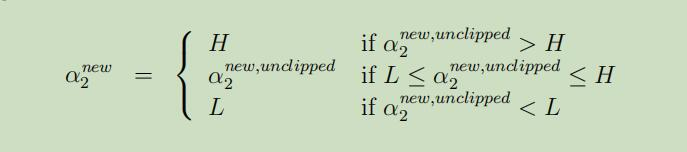然后我们可以使用最终求得的解α2带回到等式中，就可以求出最优解α1.

Repeat till convergence{
1. 选择下一步要更新的参数对αi和αj
2. 在保持其他参数都固定的情况下，使用参数αi和αj来重复优化W(x)
}


## SVM的hinge loss和梯度下降实现

### 2、SVM损失函数

SVM的损失函数就是平均合页损失函数加上L2正则,其Kernel化的推导过程如下：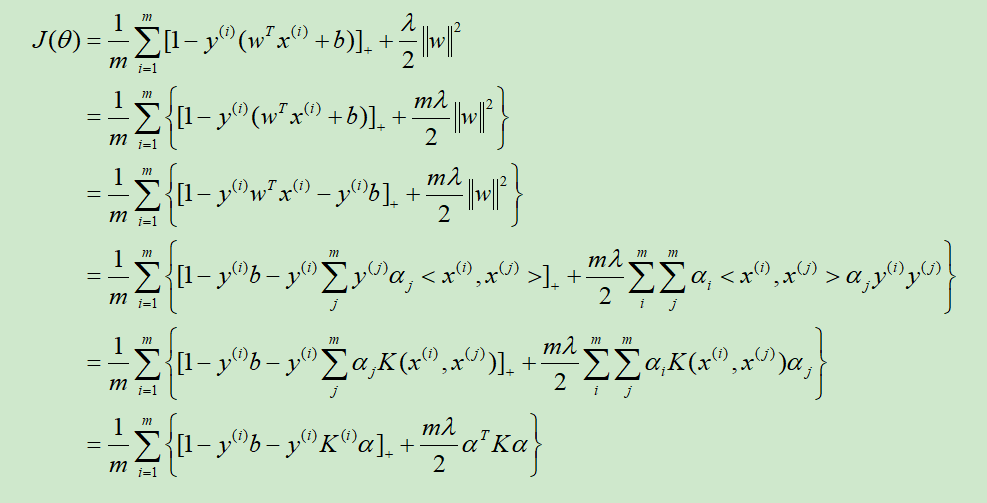### 3. 随机梯度下降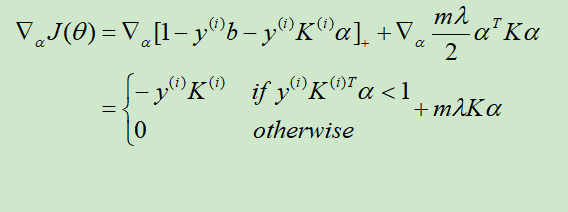注意这里NG同时还扔掉了参数b，不知道有没有影响

### 4. SVM测试